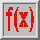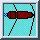# Long ranges

Up to this point, we have only considered bullets at close distances from the muzzle. We have met well-designed military projectiles and over-stabilized pistol and revolver bullets, but all of them showed dynamic stability. In other words, the maximum yaw angle, which occurs close to the muzzle, is damped out as the bullet moves on. After a traveling distance of a few thousand calibers, depending on the damping rate, the transient yaw angle practically approaches zero.

## The yaw of repose

Now let us consider a stable bullet, which has traveled a considerably longer distance. If the transient yaw has been damped out for a dynamically stable spin-stabilized projectile, does that mean that the bullet's longitudinal axis exactly coincides with the direction of movement of the CG?

It can be found from a mathematical treatment that the bullet's longitudinal axis and the direction of the velocity of the CG deviate by a small angle, which is said to be the equilibrium yaw or the yaw of repose. For right-handed spin bullets, the bullet's axis of symmetry generally points to the right and a little bit upward with respect to the direction of the velocity vector- indicating the direction into which the CG moves - , just as shown in the figure.

As an effect of this small inclination, there is a continuous air stream, which tends to deflect the bullet to the right. Thus the occurrence of the yaw of repose is the reason for bullet drift to the right (for right-handed spin) or to the left (for left-handed spin).

Usually, the yaw of repose is a very small angle and measures only fractions of a degree. The figureshows the variation of the yaw of repose angle along the trajectory for a 7.62 x 51 NATO M80 bullet fired at 32°. Although, in this example, the yaw of repose never exceeds half a degree, the resulting side drift at impact almost amounts to 100 yards.